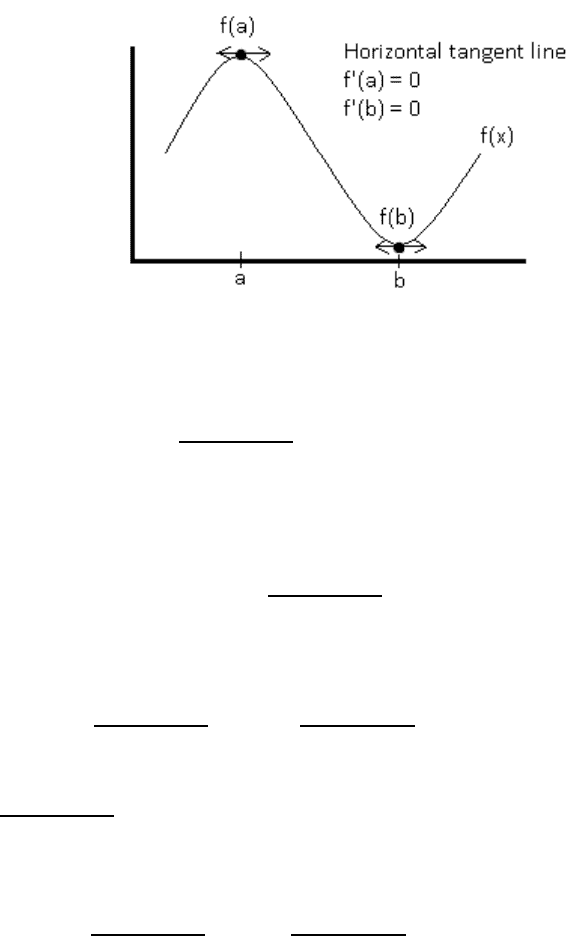# MTH 151 Study Guide - Midterm Guide: Brodmann Area 24, Differential Equation, If And Only If

47 views64 pages
School
Miami University
Department
Mathematics
Course
MTH 151
Professor
Miami University
MTH 151
Calculus I
Winter 2018
Term Test 4
Exam Guide
Unlock document

This preview shows pages 1-3 of the document.
Unlock all 64 pages and 3 million more documents.

Table of content
- Fermat’s Theorem
- Max & Min values
- Exponential Growth and Decay
- Rolle’s Theorem & the mean value theorem
- Integral test
- Optimization
- Indeterminant Forms
- Curve Sketching
- Graphing
- Antiderivatives
- Area Problem
- Fundamental theorem of calculus
- Indefinite integral
Unlock document

This preview shows pages 1-3 of the document.
Unlock all 64 pages and 3 million more documents.Fermat’s Theorem
Sketch of proof
Suppose C [a, b] is such that f(c) is a local maximum and f’(c) exists.
Since f’(c) exists, f’(c) = lim
()()
exists
If h near 0, c + h bear c. Since f(c) is a local maximum, f(c + h) f(c)
F(c + h) f(c) 0
We want to examine the sign of lim
()()
Consider left & right hand limits
h 0- : f’(c) = lim
()()
= lim
()()
lim
0 = 0
as h 0- ()()
0 since h < 0 & f(c+h) f(c) 0
therefore f’(c) 0
h 0+ , f(c) = lim
()()
= lim
()()
lim
0 = 0
f’(c) 0
Therefore since f’(c) 0 & f’(c) 0 , f’(c) = 0
Unlock document

This preview shows pages 1-3 of the document.
Unlock all 64 pages and 3 million more documents.